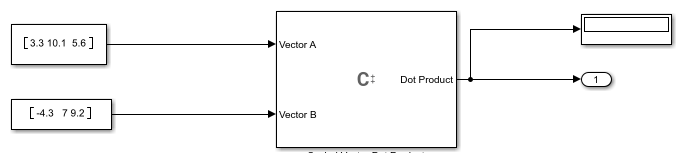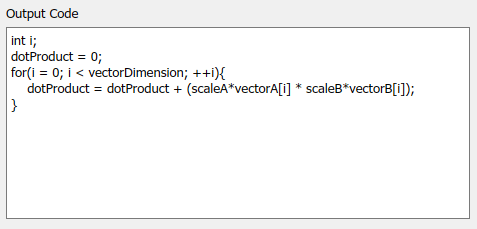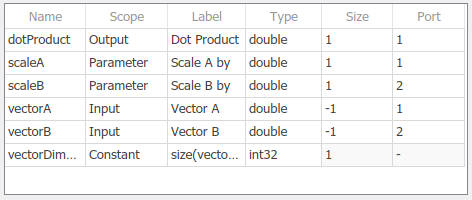# Use Inherited Sizes in a C Function Block

This example shows how to use a C Function block to compute a scaled vector dot product from two input vectors. In this example, the C Function block takes two row vectors as inputs with inherited sizes. The block scales the data using parameters. The vector dimension, which is used to define loop indices in the C code, is defined as a constant. The block calculates the dot product, and displays the results.

```open_system('CFunctionVectorDotProduct'); ```In the C Function Block Parameters dialog, the Output Code pane contains the code that performs the vector dot product calculations on the two input vectors.You must define all symbols used in the code in the Symbols table of the Block Parameters. To specify that the sizes of the input vectors are inherited, specify `-1` in the Size field of the table. The symbols `scaleA` and `scaleB` are defined as parameters of the block. These parameters appear on the block parameter mask. The definitions of all symbols used in this example are shown in the following table.